Monday 08th August 2022CBSE Guess > Papers > Important Questions > Class XI > 2010 > Maths > Maths By Mr. Anil Kumar Tondak CBSE CLASS XI
Linear Inequalities Solve the following inequalities for real x: Q.1.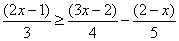Q. 2.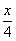<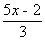-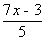Q.3.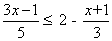Q. 4.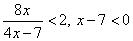Q. 5.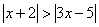Q.6.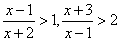Solve the following system of inequalities graphically: Q.7.Q.8.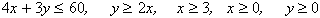Q.9. x + 2y + 3 £ 0 and x + 2y – 4 ³ 0 Q.10. x + 2y £ 10, x + y >, 1, x – y £ 0, x ³ 0, y ³ 0. Q.11. Solve the inequality, x / 4 < ( 5x – 2 ) / 3 – ( 7x – 3 ) / 5 and show the graph of the solution. Q.12. Solve the following system of inequalities graphically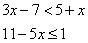and represent the solutions on the number line. Q. 13. Draw graph of the inequations 7x + 18 ³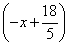and 3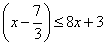Q.14. Find all pairs of consecutive odd natural numbers, both of which are larger than 10, such that their sum is less than 40. Q.15. A man wants to cut three lengths from a single piece of board of length 91 cm. The second length is to be 3 cm longer than the shortest and the third is to be twice as long as the shortest. What are the possible lengths of the shortest board if the third piece is at least 5 cm than the second. Q.16. The longest side of a triangle is 3 times the shortest side and the third side is 2 cm shorter than the longest side. If the perimeter of the triangle is at least 61 cm, find the minimum length of the shortest side. Q.17. A manufacturer has 600 litres of a 12% solution of acid. How many litres of a 30% acid solution must be added to it so that acid content in the resulting mixture will be more than 15% but less than 18%. Q.18 If a young man rides his motor cycle at 25 kph, he has to spend Rs 2 per km on petrol and if he rides it at 40 kph, the petrol cost rises to Rs 5 per km. He has to Rs 100 to spend on petrol and wishes to find the maximum distance, he can travel within one hour. Formulate the data in the form of inequation and draw a graph representing the solution of these inequation. Q.19. 150 workers were engaged to finish a job in a certain number of days. 4 workers dropped out on second day, 4 more workers dropped out on the third day and so on. It took 8 more days to finish the work. Find the number of days in which the work was completed. Q. 20. A solution of 8% boric acid is to be diluted by adding a 2% boric acid solution to it. The resulting mixture is to be more than 4% but less than 6% boric acid. If we have 640 litres of the 8% solution, how many litres of the 2% solution will have to be added? Q. 21. How many litres of water will have to be added to 1125 litres of the 45% solution of acid so that the resulting mixture will contain more than 25% but less than 30% acid content? Paper By Mr. Anil Kumar Tondak Email Id : [email protected] Ph No.: 9811363962